# 二、报表自动化范围

## 1.频率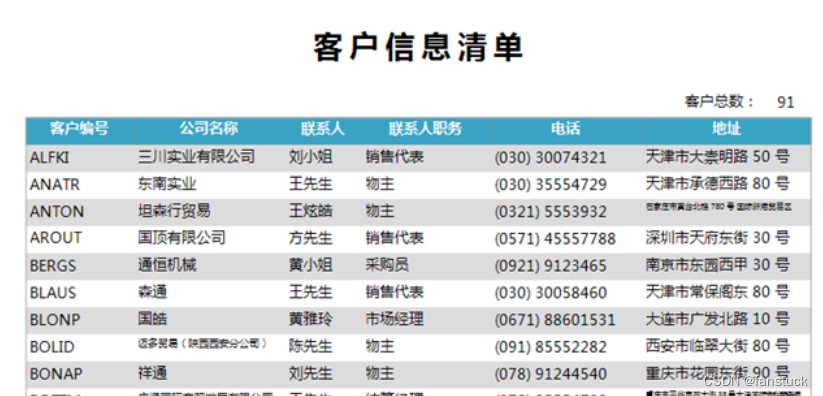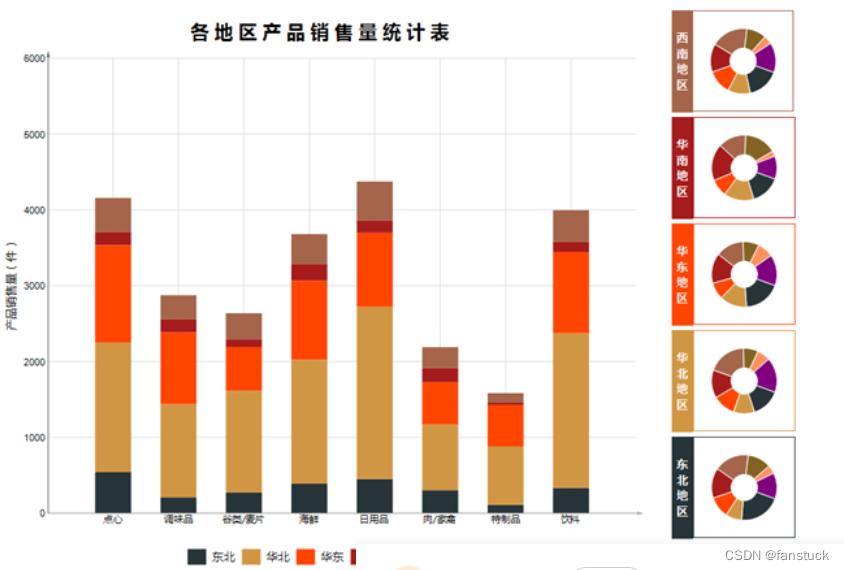## 3.流程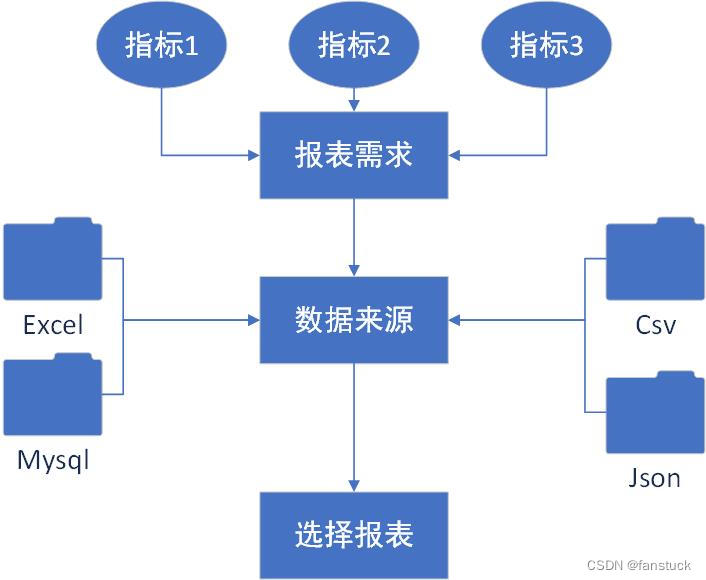# 三、实现步骤

• 指标
• 总体概览指标
反映某一数据指标的整体大小
• 对比性指标
• 同比
相邻时间段内某一共同时间点上指标的对比
• 环比
相邻时间段内的指标直接作差
• 集中趋势指标
• 平均数/加权平均数
• 众数
• 中位数
• 离散程度指标
• 全距（极差）
最大界减最小界
• 四分位数
• 方差
• 标准差
• 相关性指标
• r
•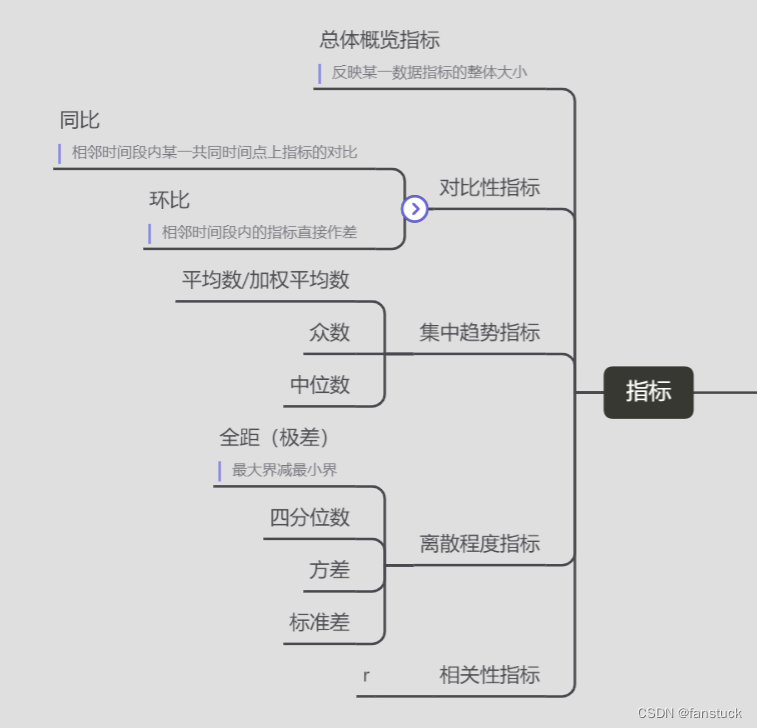我们拿一个简单的报表来进行模拟实现：

## 第一步：读取数据源文件

首先我们要了解我们的数据是从哪里来的，也就是数据源。我们最终的数据处理都是转化为DataFrame来进行分析的，所以需要对数据源进行转化为DataFrame形式：

``````import pandas as pd
import json
import pymysql
from sqlalchemy import create_engine

# 打开数据库连接
conn = pymysql.connect(host='localhost',
port=3306,
user='root',
passwd='xxxx',
charset = 'utf8'
)
engine=create_engine('mysql+pymysql://root:xxxx@localhost/mysql?charset=utf8')

return df_excel
with open(file,'r')as json_f:
return df_json
sql_cmd ='SELECT * FROM %s'%table
return df_sql
return df_csv``````

## 第二步：DataFrame计算

我们以用户信息为例：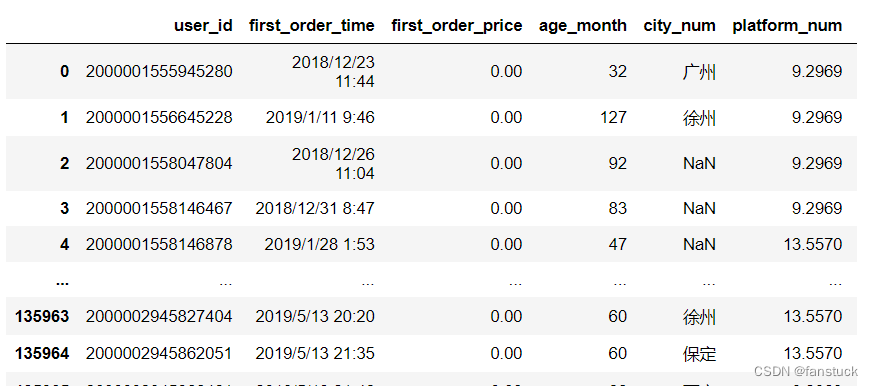我们需要统计的指标为：

#指标说明

单表图：

前十个产品受众最多的地区

产品的受众地区：

``````#将城市空值的一行删除
df=df[df['city_num'].notna()]
#删除error
df=df.drop(df[df['city_num']=='error'].index)
#统计
df = df.city_num.value_counts()``````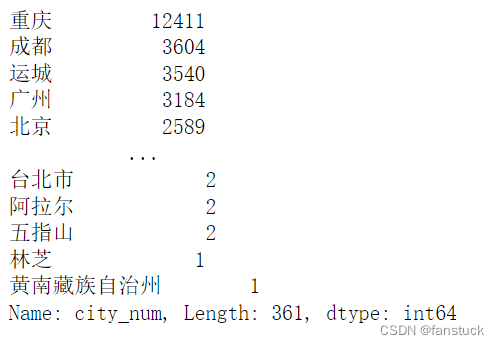我们仅获取前10名的城市就好了，封装为饼图：

``````def pie_chart(df):
#将城市空值的一行删除
df=df[df['city_num'].notna()]
#删除error
df=df.drop(df[df['city_num']=='error'].index)
#统计
df = df.city_num.value_counts()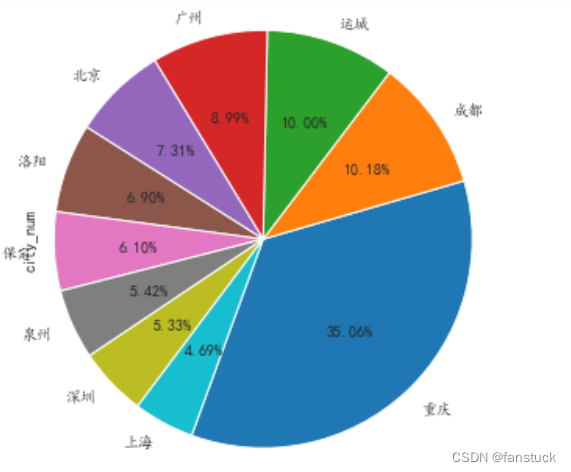将图表保存起来：

``plt.savefig('fig_cat.png')``

要是你觉得matplotlib的图片不太美观的话，你也可以换成echarts的图片，会更加好看一些：

``````
pie = Pie()
pie.set_global_opts(title_opts=opts.TitleOpts(title="前十地区"))
#pie.set_series_opts(label_opts=opts.LabelOpts(user_df))
pie.render_notebook()``````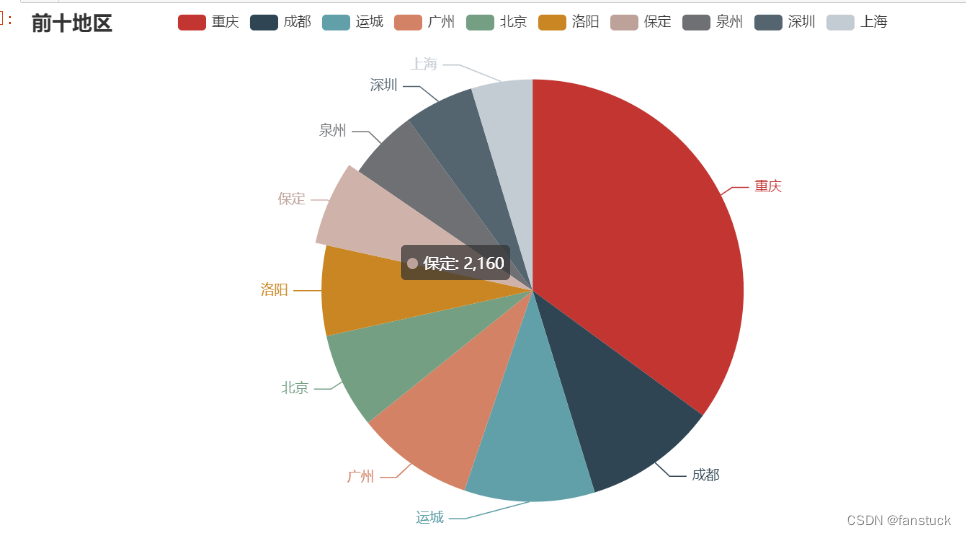封装后就可以直接使用了：

``````def echart_pie(user_df):
user_df=user_df[user_df['city_num'].notna()]
user_df=user_df.drop(user_df[user_df['city_num']=='error'].index)
user_df = user_df.city_num.value_counts()
words=list(zip(list(name),list(value)))
pie = Pie()
pie.set_global_opts(title_opts=opts.TitleOpts(title="前十地区"))
#pie.set_series_opts(label_opts=opts.LabelOpts(user_df))
return pie.render_notebook()
echart_pie(user_df)``````

可以进行保存，可惜不是动图：

``````from snapshot_selenium import snapshot
make_snapshot(snapshot,echart_pie(user_df).render(),"test.png")``````

保存为网页的形式就可以自动加载JS进行渲染了：

``````echart_pie(user_df).render('problem.html')
os.system('problem.html')``````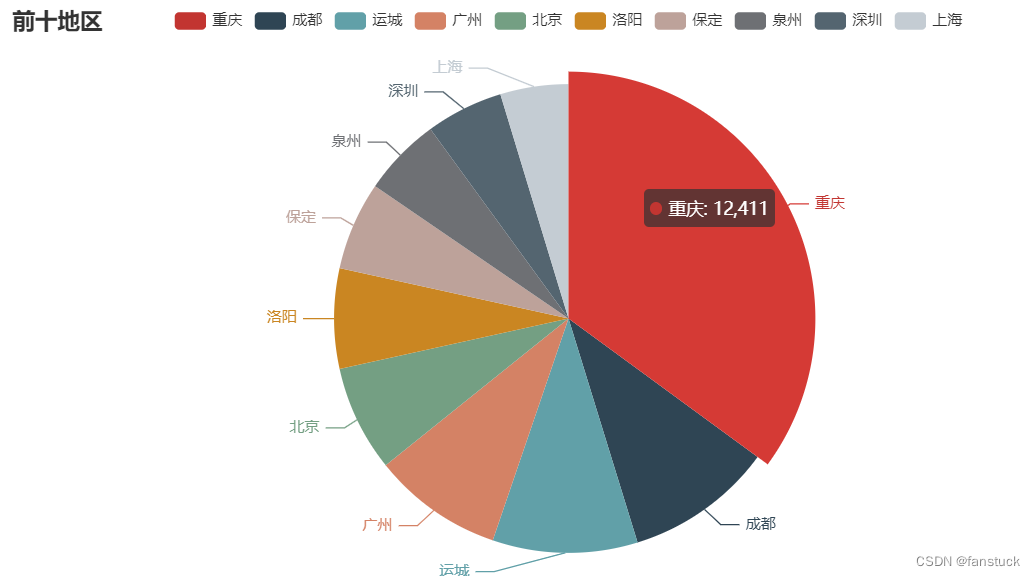## 第三步：自动发送邮件

做出来的一系列报表一般都要发给别人看的，对于一些每天需要发送到指定邮箱或者需要发送多封报表的可以使用Python来自动发送邮箱。

在Python发送邮件主要借助到smtplib和email这个两个模块。

smtplib：主要用来建立和断开与服务器连接的工作。

email：主要用来设置一些些与邮件本身相关的内容。

不同种类的邮箱服务器连接地址不一样，大家根据自己平常使用的邮箱设置相应的服务器进行连接。这里博主用网易邮箱展示：

首先需要开启POP3/SMTP/IMAP服务：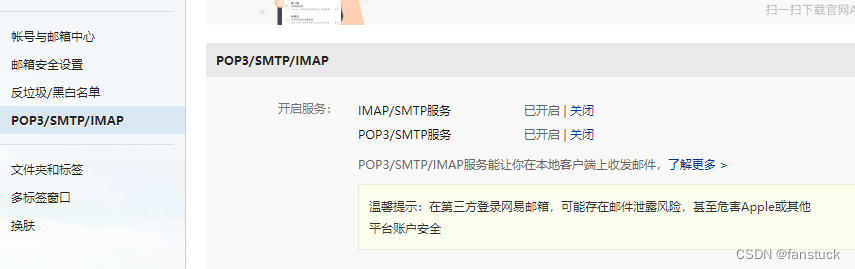之后便可以根据授权码使用python登入了。

``````import smtplib
from email import encoders
from email.mime.application import MIMEApplication
from email.mime.multipart import MIMEMultipart
from email.mime.text import MIMEText

#发件人邮箱
asender="fanstuck@163.com"
#收件人邮箱
#抄送人邮箱
acc="fanstuck@163.com"
#邮箱主题
asubject="谢谢关注"
#发件人地址
#邮箱授权码
#邮件设置
msg=MIMEMultipart()
msg['Subject']=asubject
msg['Cc']=acc
msg['from']="fanstuck"
#邮件正文
body="你好，欢迎关注fanstuck，您的关注就是我继续创作的动力！"
msg.attach(MIMEText(body,'plain','utf-8'))
#添加附件
htmlFile = 'C:/Users/10799/problem.html'

msg.attach(html)
#设置邮箱服务器地址和接口
smtp_server="smtp.163.com"
server = smtplib.SMTP(smtp_server,25)
server.set_debuglevel(1)
#登录邮箱
#发生邮箱
#断开服务器连接
server.quit()``````

运行测试：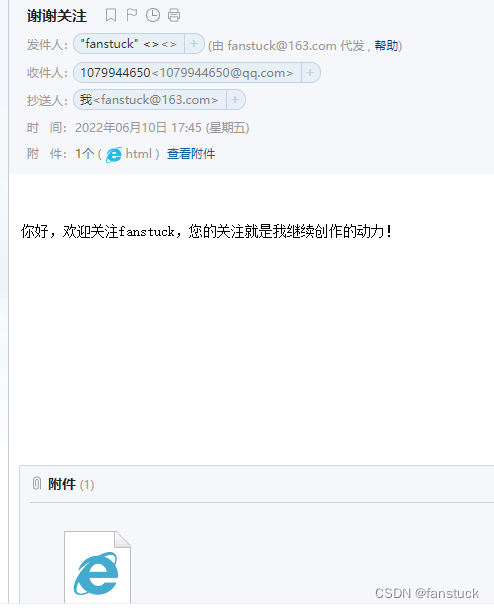下载文件：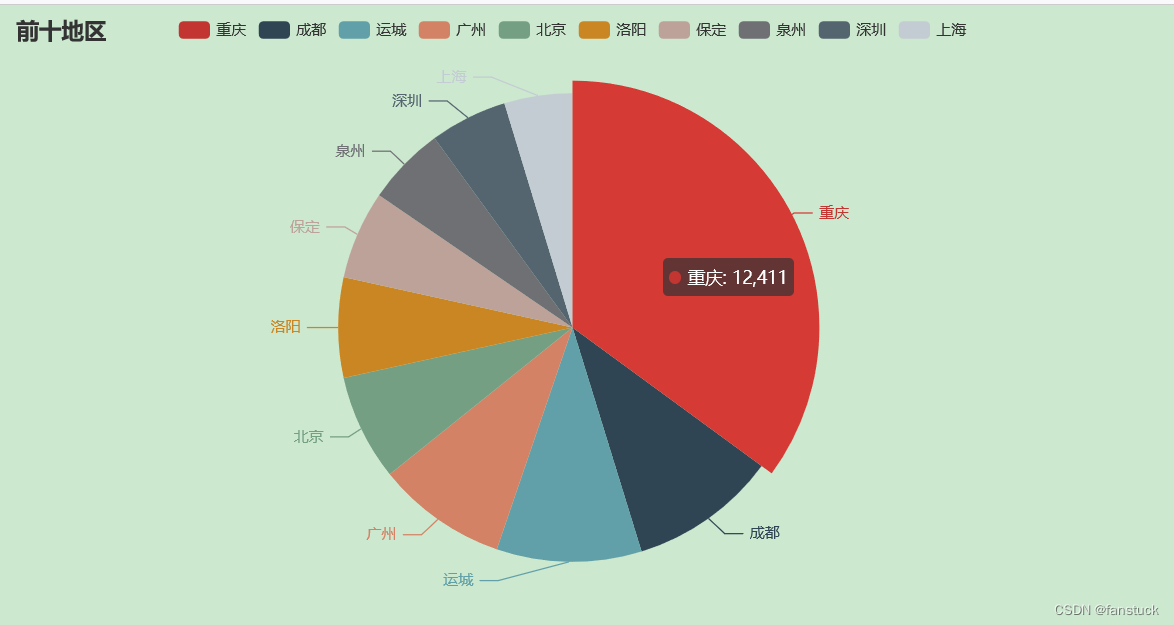完全没问题

## 点关注，防走丢，如有纰漏之处，请留言指教，非常感谢

以上就是本期全部内容。我是fanstuck ，有问题大家随时留言讨论 ，我们下期见

浅谈几种常用的报表

Python数据分析

来源：fanstuck